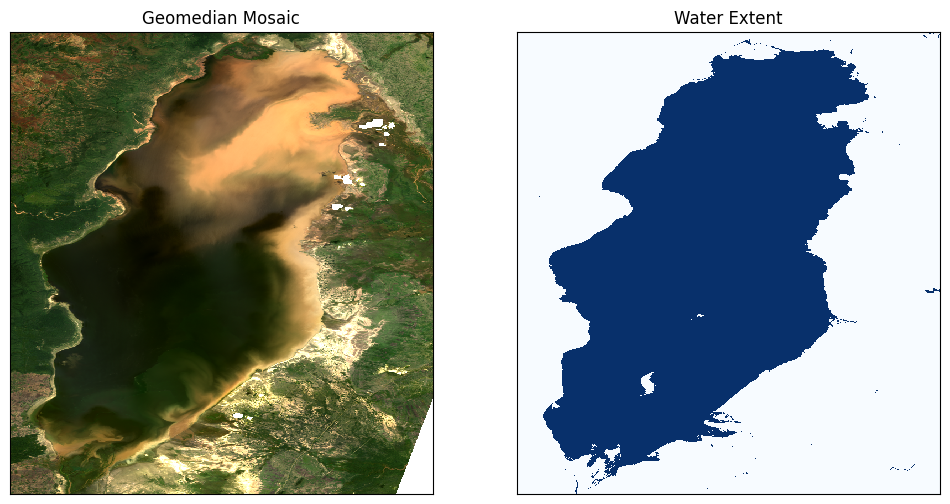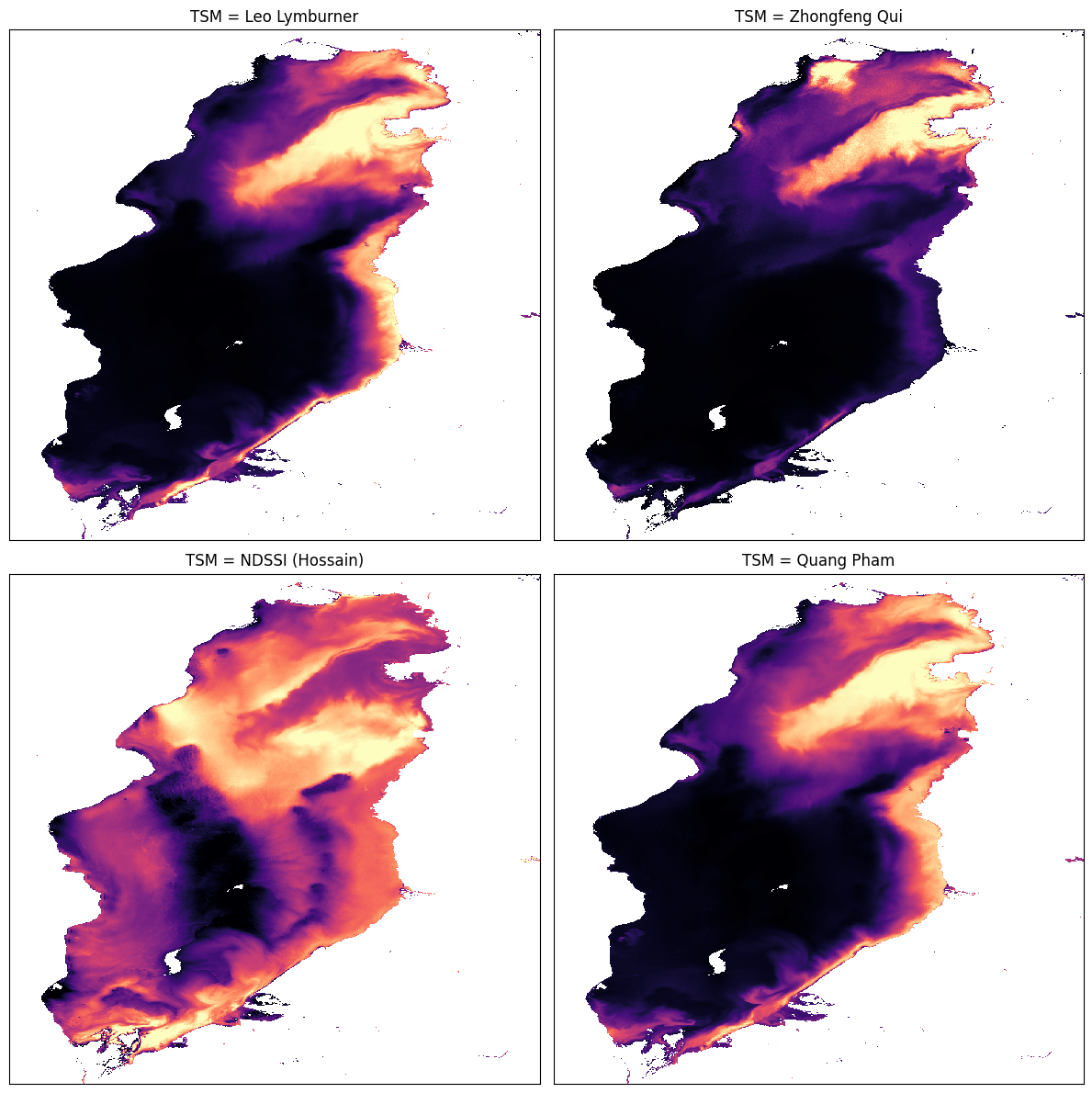# Monitoring Water Quality¶

Keywords data used; landsat 8, data used; WOfS, data methods; compositing, dask, water quality

## Contexte¶

The UN SDG 6.3.2 indicator is the « proportion of bodies of water with good ambient water quality ». There are many ways to measure water quality using remote sensing based algorithms; this notebook compares several of them. They usually estimate the amount of suspended matter in water.

## Description¶

This notebook shows results for three empirical algorithms and one spectral index addressing total suspended matter (TSM) in water. There are a number of caveats to be aware of when applying these algorithms:

• These algorithms were developed for specific regions of the world and are not necessarily universally valid.

• Landsat-8 data is a surface reflectance product, and since water has a very low radiance, the accuracy of the results can be severaly impacted by small differences in atmospheric conditions, or differences in atmospheric correction algorithms.

• The colorbars for these results have been removed to avoid showing specific quantities for the TSM results. It is best to use these results to assess qualitative changes in water quality (e.g. low, medium, high). With improvements in analysis-ready data (e.g. water leaving radiance), and with in-situ sampling of water bodies for empirical modelling, it will be possible to increase the accuracy of these water quality results and even consider the numerical output.

The showcased water quality algorithms are:

1. Lymburner Total Suspended Matter (TSM)

2. Suspended Particulate Model (SPM)

3. Normalized Difference Suspended Sediment Index (NDSSI)

4. Quang Total Suspended Solids (TSS)

## Getting started¶

To run this analysis, run all the cells in the notebook, starting with the « Load packages » cell.

Import Python packages that are used for the analysis.

:

%matplotlib inline

import datacube
import numpy as np
import xarray as xr
import matplotlib.pyplot as plt

from deafrica_tools.plotting import display_map, rgb
from deafrica_tools.datahandling import load_ard, mostcommon_crs, wofs_fuser

/usr/local/lib/python3.8/dist-packages/geopandas/_compat.py:111: UserWarning: The Shapely GEOS version (3.8.0-CAPI-1.13.1 ) is incompatible with the GEOS version PyGEOS was compiled with (3.10.1-CAPI-1.16.0). Conversions between both will be slow.
warnings.warn(


### Connect to the datacube¶

Activate the datacube database, which provides functionality for loading and displaying stored Earth observation data.

:

dc = datacube.Datacube(app="Monitoring_water_quality")


### Analysis parameters¶

The following cell sets important parameters for the analysis. The parameters are:

• lat: The central latitude to analyse (e.g. 10.338).

• lon: The central longitude to analyse (e.g. -1.055).

• lat_buffer: The number of degrees to load around the central latitude.

• lon_buffer: The number of degrees to load around the central longitude.

• time_range: The time range to analyze - in YYYY-MM-DD format (e.g. ('2016-01-01', '2016-12-31')).

If running the notebook for the first time, keep the default settings below. This will demonstrate how the analysis works and provide meaningful results. The example covers water quality in Lake Manyara.

To run the notebook for a different area, make sure Landsat 8 data is available for the chosen area using the DEAfrica Explorer. Use the drop-down menu to check Landsat 8 (ls8_sr).

#### Suggested areas¶

Here are some suggestions for areas to look at. To view one of these areas, copy and paste the parameter values into the cell below, then run the notebook.

Lake Manyara, Tanzania

lat = -3.611
lon = 35.8261
lat_buffer = 0.2025
lon_buffer = 0.1025


Weija Reservoir, Ghana

lat = 5.583
lon = -0.366
lat_buffer = 0.042
lon_buffer = 0.038


Lake Sulunga, Tanzania

lat = -6.06
lon = 35.17
lat_buffer = 0.2
lon_buffer = 0.19


### Select location¶

:

# Define the area of interest
lat = -3.611
lon = 35.8261
lat_buffer = 0.2025
lon_buffer = 0.1025

# Time period
time_range = ("2016-02-16")

# Combine central lat,lon with buffer to get area of interest
lat_range = (lat - lat_buffer, lat + lat_buffer)
lon_range = (lon - lon_buffer, lon + lon_buffer)


## View the selected location¶

The next cell will display the selected area on an interactive map. Feel free to zoom in and out to get a better understanding of the area you’ll be analysing. Clicking on any point of the map will reveal the latitude and longitude coordinates of that point.

:

# The code below renders a map that can be used to view the region.
display_map(lon_range, lat_range)

:

Make this Notebook Trusted to load map: File -> Trust Notebook

We can use the load_ard function to load data from multiple satellites (i.e. Landsat 7 and Landsat 8), and return a single xarray.Dataset containing only observations with a minimum percentage of good quality pixels.

In the example below, we request that the function returns only observations which are 90% free of clouds and other poor quality pixels by specifying min_gooddata=0.90.

:

# Create the 'query' dictionary object, which contains the longitudes,
# latitudes and time provided above
query = {
"x": lon_range,
"y": lat_range,
"time": time_range,
"resolution": (-30, 30),
"align": (15, 15),
}

# Identify the most common projection system in the input query
output_crs = mostcommon_crs(dc=dc, product='ls8_sr', query=query)

dc=dc,
products=['ls8_sr'],
measurements=["red", "green", "blue", "nir"],
group_by="solar_day",
dask_chunks={"time": 1, "x": 2000, "y": 2000},
output_crs=output_crs,
**query
)

Using pixel quality parameters for USGS Collection 2
Finding datasets
ls8_sr
Re-scaling Landsat C2 data
Returning 1 time steps as a dask array


Once the load is complete, examine the data by printing it in the next cell. The Dimensions attribute revels the number of time steps in the data set, as well as the number of pixels in the x (longitude) and y (latitude) dimensions.

:

print(ds)

<xarray.Dataset>
Dimensions:      (time: 1, y: 1498, x: 765)
Coordinates:
* time         (time) datetime64[ns] 2016-02-16T07:49:41.042103
* y            (y) float64 -3.772e+05 -3.772e+05 ... -4.22e+05 -4.221e+05
* x            (x) float64 8.025e+05 8.026e+05 ... 8.254e+05 8.254e+05
spatial_ref  int32 32636
Data variables:
red          (time, y, x) float32 dask.array<chunksize=(1, 1498, 765), meta=np.ndarray>
green        (time, y, x) float32 dask.array<chunksize=(1, 1498, 765), meta=np.ndarray>
blue         (time, y, x) float32 dask.array<chunksize=(1, 1498, 765), meta=np.ndarray>
nir          (time, y, x) float32 dask.array<chunksize=(1, 1498, 765), meta=np.ndarray>
Attributes:
crs:           EPSG:32636
grid_mapping:  spatial_ref


To make sure the algorithms for measuring water quality are only applied to areas with water, it is useful to extract the water extent from the Water Observations from Space (WOfS) product. The water extent can then be used to mask the geomedian composite so that the water quality indices are only shown for pixels that are water. Pixels that are water are selected using the condition that water == 128. To learn more about working with WOfS bit flags, see the Applying WOfS bitmasking notebook.

:

# Load water observations
product="wofs_ls",
group_by="solar_day",
like=ds.geobox,
dask_chunks={"time": 1, "x": 2000, "y": 2000},
collection_category='T1',
time=query['time']).water

#extract from mask the areas classified as water
water_extent = (water == 128).squeeze()

print(water_extent)

<xarray.DataArray 'water' (y: 1498, x: 765)>
dask.array<getitem, shape=(1498, 765), dtype=bool, chunksize=(1498, 765), chunktype=numpy.ndarray>
Coordinates:
time         datetime64[ns] 2016-02-16T07:49:41.042103
* y            (y) float64 -3.772e+05 -3.772e+05 ... -4.22e+05 -4.221e+05
* x            (x) float64 8.025e+05 8.026e+05 ... 8.254e+05 8.254e+05
spatial_ref  int32 32636

:

# Plot the geomedian composite and water extent
fig, ax = plt.subplots(1, 2, figsize=(12, 6))

#plot the true colour image
rgb(ds, ax=ax)

#plot the water extent from WOfS

# Titles
ax.set_title("Geomedian Mosaic"), ax.xaxis.set_visible(False), ax.yaxis.set_visible(False)
ax.set_title("Water Extent"), ax.xaxis.set_visible(False), ax.yaxis.set_visible(False);If the default settings were used, we should see that Lake Manyara appears to be significantly polluted in the northern half on February 16, 2016.

## Total Suspended Sediment Algorithms¶

First we will calculate the water quality using each of the algorithms, then we wil compare the approaches.

### (1) Lymburner Total Suspended Matter (TSM)¶

Paper: Lymburner et al. 2016

Units of mg/L concentration

TSM for Landsat 7:

$LYM7 = 3983 \cdot (\frac{(\text{Green} + \text{Red}) * 0.0001}{2})^{1.6246}$

TSM for Landsat 8:

$LYM8 = 3957 \cdot (\frac{(\text{Green} + \text{Red}) * 0.0001}{2})^{1.6436}$

Here, we’re using Landsat 8, so we’ll use the LYM8 function.

:

# Function to calculate Lymburner TSM value for Landsat 7
def LYM7(dataset):
return 3983 * ((dataset.green + dataset.red) * 0.0001 / 2) ** 1.6246

:

# Function to calculate Lymburner TSM value for Landsat 8
def LYM8(dataset):
return 3957 * ((dataset.green + dataset.red) * 0.0001 / 2) ** 1.6436

:

# Calculate the Lymburner TSM value for Landsat 8, using water extent to mask
lym8 = LYM8(ds).where(water_extent)

:

# Determine the 2% and 98% quantiles and set as min and max respectively
lym8_min = lym8.quantile(0.02).values
lym8_max = lym8.quantile(0.98).values


### (2) Suspended Particulate Model (SPM)¶

Units of g/m^3 concentration

SPM for Landsat 8:

$X = 2.26*(\frac{\text{Red}}{\text{Green}})^3 - 5.42*(\frac{\text{Red}}{\text{Green}})^2 + 5.58*(\frac{\text{Red}}{\text{Green}}) - 0.72$
$SPM = 10^{X} - 1.43$
:

# Function to calculate Suspended Particulate Model value
def SPM_QIU(dataset):
return (
10 ** (
2.26 * (dataset.red / dataset.green) ** 3
- 5.42 * (dataset.red / dataset.green) ** 2
+ 5.58 * (dataset.red / dataset.green)
- 0.72
)
- 1.43
)

:

# Calculate the SPM value for Landsat 8, using water extent to mask
spm_qiu = SPM_QIU(ds).where(water_extent)

:

# Determine the 2% and 98% quantiles and set as min and max respectively
spm_qiu_min = spm_qiu.quantile(0.02).values
spm_qiu_max = spm_qiu.quantile(0.98).values


### (3) Normalized Difference Suspended Sediment Index (NDSSI)¶

Paper: Hossain et al. 2010

NDSSI for Landsat 7 and 8:

$NDSSI = \frac{\text{Blue}-\text{NIR}}{\text{Blue}+\text{NIR}}$

The NDSSI value ranges from -1 to +1. Values closer to +1 indicate higher concentration of sediment.

:

# Function to calculate SNDSSI value
def NDSSI(dataset):
return (dataset.blue - dataset.nir) / (dataset.blue + dataset.nir)

:

# Calculate the NDSSI value for Landsat 8, using water extent to mask
ndssi = NDSSI(ds).where(water_extent)

:

# Determine the 2% and 98% quantiles and set as min and max respectively
ndssi_min = ndssi.quantile(0.02).compute().values
ndssi_max = ndssi.quantile(0.98).compute().values


### (4) Quang Total Suspended Solids (TSS)¶

Paper: Quang et al. 2017

Units of mg/L concentration

$Quang8 = 380.32 * \text{Red}*0.0001 - 1.7826$
:

# Function to calculate quang8 value
def QUANG8(dataset):
return 380.32 * (dataset.red) * 0.0001 - 1.7826

:

# Calculate the quang8 value for Landsat 8, using water extent to mask
quang8 = QUANG8(ds).where(water_extent)

:

# Determine the 2% and 98% quantiles and set as min and max respectively
quang8_min = quang8.quantile(0.02).compute().values
quang8_max = quang8.quantile(0.98).compute().values


## Compare the algorithms” outputs¶

:

fig, ax = plt.subplots(2, 2, figsize=(12, 12))
cmap='magma'

lym8.plot(
ax=ax[0, 0], cmap=cmap, add_colorbar=False, vmin=lym8_min, vmax=lym8_max
)

spm_qiu.plot(
ax=ax[0, 1], cmap=cmap, add_colorbar=False, vmin=spm_qiu_min, vmax=spm_qiu_max
)

ndssi.plot(
ax=ax[1, 0], cmap=cmap, add_colorbar=False, vmin=ndssi_min, vmax=ndssi_max
)

quang8.plot(
ax=ax[1, 1], cmap=cmap, add_colorbar=False, vmin=quang8_min, vmax=quang8_max
)

print(
"Total Suspended Matter (Black=Low, Purple=Medium-Low, "
"Orange=Medium-High, Yellow=High)"
)

# Titles
ax[0, 0].set_title("TSM = Leo Lymburner"), ax[0, 0].xaxis.set_visible(False), ax[0, 0].yaxis.set_visible(False)
ax[0, 1].set_title("TSM = Zhongfeng Qui"), ax[0, 1].xaxis.set_visible(False), ax[0, 1].yaxis.set_visible(False)
ax[1, 0].set_title("TSM = NDSSI (Hossain)"), ax[1, 0].xaxis.set_visible(False), ax[1, 0].yaxis.set_visible(False)
ax[1, 1].set_title("TSM = Quang Pham"), ax[1, 1].xaxis.set_visible(False), ax[1, 1].yaxis.set_visible(False)

plt.tight_layout();

Total Suspended Matter (Black=Low, Purple=Medium-Low, Orange=Medium-High, Yellow=High)If the default settings were used, we should see that all the indices reflect the appearance of the lake (where murky, the index is high) except NDSSI, though it does appear to be related to the others - mostly low where the others are high and vice versa.

Contact: If you need assistance, please post a question on the Open Data Cube Slack channel or on the GIS Stack Exchange using the open-data-cube tag (you can view previously asked questions here). If you would like to report an issue with this notebook, you can file one on Github.

Compatible datacube version:

:

print(datacube.__version__)

1.8.7


Last Tested:

:

from datetime import datetime
datetime.today().strftime('%Y-%m-%d')

:

'2022-06-22'